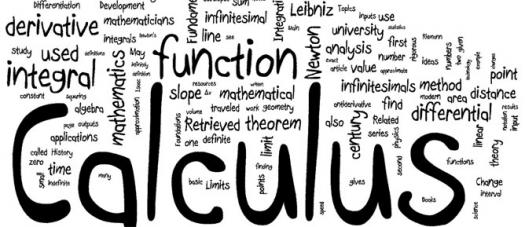# Volume Using Calculus Assessment Test

10 Questions | Total Attempts: 108SettingsTake our intelligent assessment test to evaluate your knowledge of how we can use calculus and integrals to find volumes like areas and lengths.

• 1.
An integral over a 3-dimensional domain is referred to as?
• A.

Slope

• B.

Volume integral

• C.

Space

• D.

Function

• 2.
The amount of space taken up by a three-dimensional object is?
• A.

Volume

• B.

Time

• C.

Length

• D.

Option

• 3.
How do you find the volume of a solid?
• A.

Length×length

• B.

Length×width×height

• C.

• D.

Length×height

• 4.
The quantity of three-dimensional space enclosed by a closed surface is?
• A.

Term

• B.

Vacuum

• C.

Volume

• D.

Area

• 5.
What is the unit of volume?
• A.

Time

• B.

Second

• C.

Cubic metre

• D.

Metre

• 6.
The amount that something can hold is termed?
• A.

Figure

• B.

Capacity

• C.

Power

• D.

Number

• 7.
Which is an important parameter in calculating the volume of an object with density?
• A.

Mass

• B.

Time

• C.

Height

• D.

Figure

• 8.
Which is an important parameter in finding the volume of a pyramid?
• A.

Level

• B.

Height

• C.

Time

• D.

Space

• 9.
How do you find the volume of a triangular pyramid?
• A.

1/3AH

• B.

1/2B

• C.

3AB

• D.

2BC

• 10.
How do you calculate the volume of a cylinder?
• A.

πr

• B.

πr2

• C.

πr^2

• D.

πr2h

Related TopicsBack to top